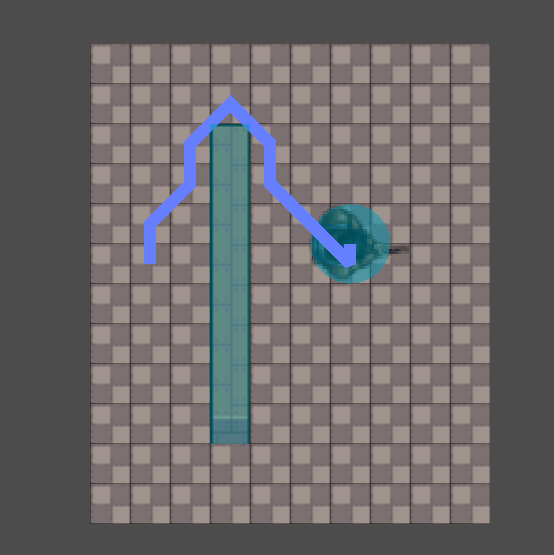Map is 2d top-down composed of TileMap with two tile types: wall and floor. Walls have a square collision polygon, floor tiles are just sprites. Tile cell size is 16x16px.

Player is a KinematicBody2D, containing circle CollisionShape2D of 16px radius, with point and click movement. Pathfinding is done through TileMap by AStar class.

Issue is that path Point can be 8px close to the Wall and Player center is 16px away from Wall. I solved this issue by calculating Plane from collision point and normal and
moving Point away from Wall. Now moving parallel to the Wall is more or less fine. But this doesn't solve moving around corner and over top of the Wall. The collision normal
in this points are weirdly angled and doesn't correspond with Wall surface normal Player hitting. This makes Player "bounce" up and down moving around the thin Wall bits.

So my question is if there is a way to get surface normal of collided Wall? Or may be I'm getting wrong way about this and there is a completely different solution to my whole problem?``````func move_along(delta):
var point = get_point()
if !point: return
if position.distance_to(point) < 1:
next_point()
return

var velocity = (point - position).normalized() * move_speed
var collision = move_and_collide(velocity * delta)

if collision:
# Distance from the Origin to the collision Plane
var D = collision.normal.dot(collision.position)
# distance from Point to Plane
var ptp = collision.normal.dot(point) - D
# Offset - how far to move Point from the Plane:
# distance from Body center to Plane minus distance from Point to Plane
var offset = position.distance_to(collision.position) - ptp
var offset_vector = collision.normal * (offset + 0.1)
var new_point = offset_vector + point

if ptp > 0:
next_point(new_point)
else: